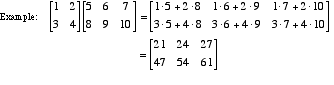# matrix multiplication

Matrix Multiplication

Matrices are multiplied by the system shown below. Matrices may not be multiplied unless their dimensions are compatible. Note: When an a × b matrix is multiplied times a b × c matrix, the product has dimensions a × c.